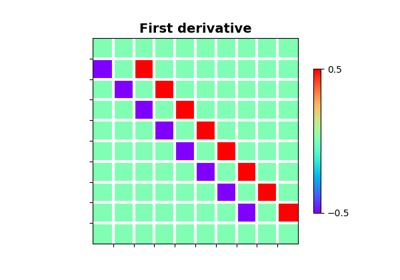Apply gradient operator to a multi-dimensional array.

Note

At least 2 dimensions are required, use pylops.FirstDerivative for 1d arrays.

Parameters
dimstuple

Number of samples for each dimension.

samplingtuple, optional

Sampling steps for each direction.

edgebool, optional

Use reduced order derivative at edges (True) or ignore them (False).

kindstr, optional

Derivative kind (forward, centered, or backward).

dtypestr, optional

Type of elements in input array.

Returns
l2oppylops.LinearOperator

Notes

The Gradient operator applies a first-order derivative to each dimension of a multi-dimensional array in forward mode.

For simplicity, given a three dimensional array, the Gradient in forward mode using a centered stencil can be expressed as:

$\mathbf{g}_{i, j, k} = (f_{i+1, j, k} - f_{i-1, j, k}) / d_1 \mathbf{i_1} + (f_{i, j+1, k} - f_{i, j-1, k}) / d_2 \mathbf{i_2} + (f_{i, j, k+1} - f_{i, j, k-1}) / d_3 \mathbf{i_3}$

which is discretized as follows:

$\begin{split}\mathbf{g} = \begin{bmatrix} \mathbf{df_1} \\ \mathbf{df_2} \\ \mathbf{df_3} \end{bmatrix}\end{split}$

## Examples using pylops.Gradient#